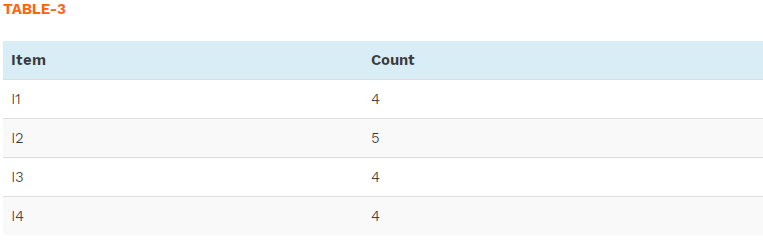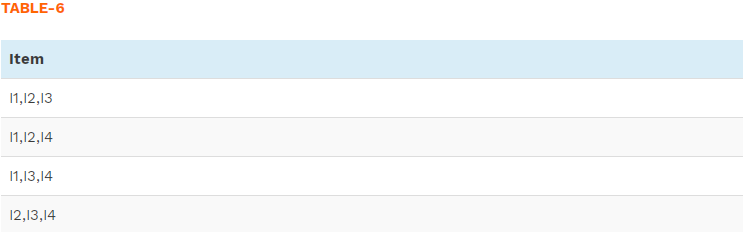top of page
Search

# Apriori algorithmTo better understand this concept we will first discuss a few very common examples.

The famous Wal-Mart’s beer and diaper parable: A sales person from Wal-Mart trying to increase the sales of the store analysed all sales records. He discovered a general trend that people who buy diapers often buy beers as well. Although the two products are unrelated this relation arises because parenting is a difficult task for many. By bundling the products together and giving discounts on them the sale person was able to escalate the sales.

Likewise, you may have seen in supermarkets that bread and jams are placed together, which makes it easy for a customer to find them together and thereby increasing the chance of purchasing both the items.

Also, you must have noticed that the local vegetable seller always bundles onions and potatoes together. He even offers a discount to people who buy these bundles. He does so because he realizes that people who buy potatoes also buy onions. Therefore, by bunching them together, he makes it convenient for the customers. At the same time, he also increases his sales performance. It also allows him to offer discounts.

Moreover, apart from the field of sales, in medical diagnosis for instance, understanding which symptoms tend to co-morbid can help to improve patient care and medicine prescription.

The above mentioned are examples of Association rules in data mining. It will help us get an idea about the Apriori algorithm.To generalise the above examples, if there is a pair of items, A and B (or more), that are frequently bought together:

• Both A and B can be placed together on a shelf, so that buyers of one item would most probably buy the other.

• Promotional discounts could be applied to just one out of the two items.

• A and B could be combined into a new product, such as having B in flavours of A.

To uncover such associations we make use of Association rules in data mining.

Now we will define some concepts which will aid us in understanding the Apriori algorithm.

## Association rule mining:

Association Rule Mining is used when we want to discover an association between different objects in a set, find frequent patterns in a transaction database, relational databases or any other information repository. It tells us what items do customers frequently buy together by generating a set of rules called Association Rules. To put it simply, it gives you output as rules in form if this then that.

A formal definition of the problem of association rules given by Rakesh Agrawal, the President and Founder of the Data Insights Laboratories:

Let I = {i1, i2, i3, …, in} be a set of n attributes called items and D={t1, t2, …, tn} be the set of transactions. It is called database. Every transaction, ti in D has a unique transaction ID, and it consists of a subset of itemsets in I.

A rule can be defined as an implication, X⟶Y where X and Y are subsets of I(X, Y⊆ I), and they have no element in common, i.e., X∩Y. X and Y are the antecedent and the consequent of the rule, respectively.

Let us take a look at an example.

Consider the following database, where each row is a transaction and each cell is an individual item of the transaction:The association rules that can be determined from this database are the following:

1. 100% of sets with alpha also contain beta

2. 50% of sets with alpha, beta also have epsilon

3. 50% of sets with alpha, beta also have theta

There are three common ways to measure association:

1. Support: refers to items’ frequency of occurrence. It tells how popular an itemset is, as measured by the proportion of transactions in which an itemset appears.

supp (X) = Number of transaction in which X appears / Total number of transactions

2. Confidence: It is a conditional probability. It tells us how likely item Y is purchased when item X is purchased, it is expressed as {X -> Y}. This is measured by the proportion of transactions with item X, in which item Y also appears.

conf (X⟶Y) = supp (X ∪ Y) / supp (X)

But there is a major drawback. It only takes into account the popularity of the itemset X and not the popularity of Y. If Y is as popular as X then there will be a higher probability that a transaction containing X will also contain Y thus increasing the confidence. To overcome this drawback there is another measure called lift.

3. Lift: It signifies the likelihood of the itemset Y being purchased when item X is purchased while taking into account the popularity of Y.

lift ( X⟶Y) = supp (X ∪ Y) / supp (X) ∗ supp (Y)

A lift value greater than 1 means that item Y is likely to be bought if item X is bought, while a value less than 1 means that item Y is unlikely to be bought if item X is bought.## Frequent Itemset:

Itemset: A set of items together is called an itemset. If any itemset has k-items it is called a k-itemset. An itemset consists of two or more items.

An itemset that occurs frequently is called a frequent itemset.

A set of items is called frequent if it satisfies a minimum threshold value for support and confidence. Support shows transactions with items purchased together in a single transaction. Confidence shows transactions where the items are purchased one after the other.

We will now discuss Apriori algorithm.

## Apriori Algorithm

Definition: It is an algorithm for frequent itemset mining and association rule learning over relational databases. It proceeds by identifying the frequent individual items in the database and extending them to larger and larger item sets as long as those item sets appear sufficiently often in the database. The frequent item sets determined by Apriori can be used to determine association rules which highlight general trends in the database.

It was proposed by R. Agrawal and R. Srikant in 1994 for finding frequent itemsets in a dataset for boolean association rule. Name of the algorithm is Apriori because it uses prior knowledge of frequent itemset properties.

A major concept in Apriori algorithm is the anti-monotonicity of the support measure. It assumes that

1. All subsets of a frequent itemset must be frequent

2. Similarly, for any infrequent itemset, all its supersets must be infrequent too

Apriori uses a "bottom up" approach, where frequent subsets are extended one item at a time (a step known as candidate generation), and groups of candidates are tested against the data. The algorithm terminates when no further successful extensions are found.

This algorithm uses two steps “join” and “prune” to reduce the search space. It is an iterative approach to discover the most frequent itemsets. The steps followed in the Apriori Algorithm of data mining are:

1. Join Step: This step generates (K+1) itemset from K-itemsets by joining each item with itself.

2. Prune Step: This step scans the count of each item in the database. If the candidate item does not meet minimum support, then it is regarded as infrequent and thus it is removed. This step is performed to reduce the size of the candidate itemsets.

### Steps In Apriori:

This algorithm follows a sequence of steps to find the most frequent itemset in the given database. It follows the join and the prune steps iteratively until the most frequent itemset is achieved. A minimum support threshold is given in the problem or it is assumed by the user.Step 1: In the first iteration of the algorithm, each item is taken as a 1-itemset candidate. The algorithm will count the frequency of each item.

Step 2: Let the minimum support be assigned ( e.g. 2). The set of 1 – itemset whose frequency is satisfying the minimum support are determined. Only those candidates which count more than or equal to minimum support, are taken ahead for the next iteration and the others are pruned.

Step 3: Then, 2-itemset frequent items with minimum support are discovered. For this step, the 2-itemset is generated by forming a group of 2 by combining items with itself (join step).

Step 4: The 2-itemset candidates are pruned using minimum support threshold value. Now the table will have 2 –itemsets with minimum support only.

Step 5: The next iteration will form 3 –itemsets using join and prune step. This iteration will follow anti-monotone property where the subsets of 3-itemsets, that is the 2 –itemset subsets of each group fall in minimum support. If all 2-itemset subsets are frequent then the superset will be frequent otherwise it is pruned.

Step 6: Next step will make 4-itemset by joining 3-itemset with itself and pruning if its subset does not meet the minimum support criteria. The algorithm is stopped when the most frequent itemset is achieved.

Let us discuss an example to understand these steps more clearly.

Consider the following table:We will generate the association rules for the above table using Apriori algorithm.

Let the minimum support be 3.

Step 1: Create the frequency distribution table of the items.Step 2: This is prune step, the item whose count does not meet minimum support are removed. Only I5 item does not meet minimum support of 3, thus it is removed. Items I1, I2, I3, I4 meet minimum support count and therefore they are frequent.Step 3: This is a join step, here we will generate a list of all pairs of the frequent items (2-itemset).Step 4: This is a prune step. The pairs I1,I4 and I3I4 do not meet the minimum support and are removed.Step 5: This is a join and prune step, here we will now look for frequent triples in the database, but we can already exclude all the triples that contain pairs which were not frequent in the previous step (following the anti-monotone property of support measure).

For example, in the table below:We can see for itemset {I1, I2, I4}, the subsets are: {I1, I2}, {I1, I4}, {I2, I4}.

The pair {I1, I4} is not frequent, as it do not occur in TABLE-5. Thus {I1, I2, I4} is not frequent, hence it is deleted.

Similarly, all the triples are pruned in this way and we are left only the itemset {I1, I2, I3} which is frequent as all its subsets are frequent (again the anti-monotone property of support measure comes into play here).

Step 6: Generate Association Rules: From the frequent itemset discovered above and setting the minimum confidence threshold to be 60%, the association could be:

{I1, I2} => {I3}

Confidence = support {I1, I2, I3} / support {I1, I2} = (3/ 4)* 100 = 75%

{I1, I3} => {I2}

Confidence = support {I1, I2, I3} / support {I1, I3} = (3/ 3)* 100 = 100%

{I2, I3} => {I1}

Confidence = support {I1, I2, I3} / support {I2, I3} = (3/ 4)* 100 = 75%

{I1} => {I2, I3}

Confidence = support {I1, I2, I3} / support {I1} = (3/ 4)* 100 = 75%

{I2} => {I1, I3}

Confidence = support {I1, I2, I3} / support {I2 = (3/ 5)* 100 = 60%

{I3} => {I1, I2}

Confidence = support {I1, I2, I3} / support {I3} = (3/ 4)* 100 = 75%

This shows that all the above association rules are strong if minimum confidence threshold is 60%.